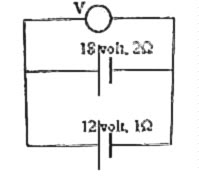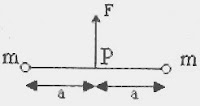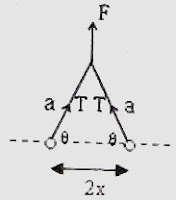## Thursday, August 30, 2007

### Multiple Choice Questions on Direct Current CircuitsThe following MCQ which appeared in All India Pre-Medical/Dental Entrance 2005 Examination (AIPMT) is one requiring the application of Kirchoff’s laws:

Two batteries, one of emf 18 volt and internal resistance 2 Ω and the other of emf 12 volt and internal resistance 1 Ω, are connected as shown. The voltmeter V will record a reading of

(a) 30 volt (b) 18 volt (c) 15 volt (d) 14 volt

The net emf in the closed path containing the two batteries is 18volt – 12 volt = 6 volt

Since this must be equal to the net voltage drop across the internal resistances of the batteries, we have

I(2 + 1) = 6 volt where ‘I’ is the current through the batteries. [Note that the voltmeter will draw negligible current (ideally, zero current) and hence the same current flows through the batteries].

Therefore, I = 2 ampere.

The voltage across the voltmeter is the same as the terminal voltages of the batteries. If you consider the 18 volt battery, its terminal voltage is 18 – (2×2) = 14 volt.

[If you consider the 12 volt battery, its terminal voltage is 12 + (1×2) = 14 volt, which is the same as the above value]. So, the correct option is (d).

Here is another question which you can answer in no time if you had worked out a similar one earlier:

Five identical cells each of emf 1.5 V and internal resistance ‘r’ send the same current through an external resistance of 1 Ω whether the cells are connected in series or in parallel. The internal resistance ‘r’ of each cell is

(a) 0.2Ω (b) 0.5 Ω (c) 1 Ω (d) 1.5 Ω (e) 3 Ω

The condition depicted in the above question occurs when the internal resistance of each cell is equal to the external resistance. The answer therefore is 1 Ω.

The proof is simple: Let there be ‘n’ cells. If the emf of each cell is V and the external resistance is R, the current through R on connecting the cells in series is nV/(nr + R). When the cells are in parallel, the current through R is V/[(r/n) + R].

Since the currents are equal, nV/(nr + R) = V/[(r/n) + R].

Therefore, r + nR = nR + R, from which r = R.

Now, let us consider the following MCQ which appeared in All India Pre-Medical/Dental Entrance 2004 Examination question paper:

Five equal resistances each of resistance R are connected as shown in the figure. A battery of V volts is connected between A and B. The current flowing in AFCEB will be(a) 3V/R (b) V/R (c) V/2R (d) 2V/R

Once you realise that C and D are equipotential points with respect to point A, the resistance R connected directly between C and D can be ignored (since it will not carry any current). The current through the path AFCEB is therefore V/2R.

`Science is a wonderful thing if one does not have to earnone's living at it`
`–Albert Einstein `

## Saturday, August 25, 2007

### Two Questions (MCQ) on Capacitors

Here are two multiple choice questions which will be interesting to you:

(1) Six identical capacitors C1, C2, C3, C4, C5 and C6 each having capacitance C are connected as shown. The equivalent capacitance between the points A and B is

(a) C (b) 2C (c) 2.5C (d) 3C (e) 3.5CThis may appear to be a difficult question for some of you. In questions of this type try to identify equipotential points. Circuit elements connected between equipotential points can be ignored. In most cases the circuit will then become simple.

In the present case, the junction between C1 and C6 is at the same potential as that at the junction between C2 and C5. [This follows since the capacitors are identical. Generally, the points will be equipotential points if the balance condition for Wheatstone bridge is satisfied: C1/C6 = C2/C5]. The capacitor C3 connected between the equipotential points can therefore be ignored.

Now we have three parallel connections across the points A and B:

(i) Series combination of C1 and C6 giving a capacitance C/2.

(ii) Series combination of C2 and C5 giving a capacitance C/2.

(iii) Capacitor C4 of value C.

The parallel combined value of the abve three connections is (C/2) + (C/2) + C = 2C.

(2) In a circuit, capacitors C1 and C2 (in series) are connected between two points A and B (Fig). If the potentials at A and B are V1 and V2 respectively, what is the potential at the junction point P between C1 and C2?

(a) C1(V1+V2)/(C1+C2)

(b) (V1 + V2)/2

(c) V1 V2

(d) (C1V2 + C2V1)/ (C1 + C2)

(e) (C1V1 + C2V2)/ (C1 + C2)

Since the capacitors are in series, they carry the same charge. If the potential at the junction point P is ‘V’, the charges on the capacitors C1 and C2 are respectively C1(V – V1) and C2(V2 – V).

Therefore, we have

C1(V – V1) = C2(V2 – V), from which

V = (C1V1 + C2V2)/ (C1 + C2).

You can find more posts on electrostatics by clicking on the label ELECTROSTATICS below this post or on the left side of this page.

## Monday, August 20, 2007

### Three KEAM 2007 Multiple Choice Questions from Heat and Thermodynamics

Four questions from Heat and Thermodynamics appeared in the Kerala Engineering Entrance 2007 question paper. One question was quite simple, meant for testing your knowledge of the temperature at which water has minimum volume, which most of you know, is 4º C. Here are the remaining three questions:
(1) A closed gas cylinder is divided into two parts by a piston held tight. The pressure and volume of gas in two parts respectively are (P, 5V) and (10 P, V). If now the piston is left free and the system undergoes isothermal process, then the volume of the gas in two parts respectively are
(a) 2 V, 4 V (b) 3 V, 3 V (c) 5 V, V (d) 4 V, 2 V (e) 2.5 V, 3.5 V
Since the temperature is constant (isothermal process), we can apply Boyle’s law. The piston will move towards the lower pressure side until the pressures on the two sides are the same. If this common pressure is P’, we have
P×5V + 10P×V = P’×6V, since the total volume is 6 V.
From this, P’ = (15/6) P.
Now, applying Boyle’s law to the gas on the two sides separarely, we have
P×5V = (15/6) P×V1 and
10P×V = (15/6) P×V2
where V1 and V2 are the volumes of the gas in the two parts respectively.
From the above equations, we obtain V1 = 2V and V2 = 4V [Option (a)].
(2) A Carnot engine with sink’s temperature at 17º C has 50% efficiency. By how much should its source temperature be changed to increase its efficiency to 60%?
(a) 225 K (b) 128º C (c) 580 K (d) 145 K (e) 145º C
We have efficiency η = (T1 T2)/T1 where T1 and T2 are the temperatures of the source and sink respectively.
Here, η = 0.5 and T2 = 17º C = 290 K. On substituting these values, we obtain
1– (290/T1) = 0.5, from which T1 = 580 K.
When the efficiency is 60%, we have
1– (290/T1') = 0.6, from which T1' = 725 K.
Therefore, the change in source temperature = 725 – 580 = 145 K.
(3) Two moles of oxygen is mixed with eight moles of helium. The effective specific heat of the mixture at constant volume is
(a) 1.3 R (b) 1.4 R (c) 1.7 R (d) 1.9 R (e) 1.2 R
Oxygen is diatomic and hence its molar specific heat at constant volume is (5/2) R (corresponding to 5 degrees of freedom).
Helium is mono-atomic and hence its molar specific heat at constant volume is (3/2) R (corresponding to 3 degrees of freedom).
Therefore, the total quantity of heat required to raise the temperature of two moles of oxygen and eight moles of helium through 1 K is
2×(5/2)R + 8×(3/2)R = 17 R.
Since the number of moles in the mixture is 10, the effective molar specific heat of the mixture is (17/10)R = 1.7 R.

## Friday, August 10, 2007

### Solution to MCQ on Friction

In the post dated 9th August 2007, two questions on friction were given without solution. These questions with solution are given below as promised in the post:

(1) A block of mass ‘M’ equal to 1 kg is resting on a frictionless horizontal surface. Another block of mass ‘m’ equal to 0.2 kg is resting on the top surface of the 1 kg block. The coefficient of friction between the two blocks is 0.2. The motion of the 1 kg kg block is controlled by a light spring of force constant 24 Nm–1 arranged as shown in the figure. What is the maximum amplitude of oscillation of the blocks (together) so that the upper block does not slip relative to the lower block? (g = 10 ms–2)

(a) 2 cm (b) 5 cm (c) 10 cm (d) 20 cm (e)50cm

The figure is not given here. You will find it with the post dated 9th August 2007 by moving a little down on this page.

If ‘x’ is the maximum possible amplitude, the maximum restoring force exeretred by the spring is Kx and the maximum acceleration of the two blocks (while moving together) is Kx/(M+m).

The force experienced by the upper block while moving with the above acceleration is mKx/(M+m). The upper block will just start slipping when this force exceeds the frictional force μmg where ‘μ’ is the coefficient of friction so that in the limiting case, we have

mKx/(M+m) = μmg.

Therefore, x = μg(M+m)/K = 0.2×10(1+0.2)/24 = 0.1 m = 10 cm.

(2) A metallic cube takes ‘t’ seconds to slide down a smooth inclined plane of angle 45º. When the inclined plane is made rough, the block takes nt seconds to slide down. The coefficient of friction between the block and the rough incline is

(a) 1/n (b) 1 (1/n) (c) 1 (1/n2) (d) (1/n2) (e) 1 + (1/n2)

In the case of the smooth inclined plane, the acceleration of the block down the plane is gsinθ where ‘θ’ is the angle of the plane (equal to 45º). If ‘s’ is the length of the plane, we have

s = 0 + ½ (gsinθ)t2

[We have used the equation of linear motion, s = ut + ½ at2]

In the case of the rough inclined plane, since the net force acting on the block is (mgsinθ μmgcosθ), the acceleration of the block down the plane is g(sinθ μcosθ).

Therefore, we have

s = 0 + ½ g(sinθ μcosθ) (nt)2

From the above equations, we obtain

sinθ(n2 – 1) = μn2cosθ

Therefore, μ = [(n2 – 1) /n2] tanθ.

Since θ = 45º, tanθ =1 and hence μ = (n2 – 1)/n2 = 1 (1/n2)

## Thursday, August 09, 2007

### Two Practice Problems (MCQ) on Friction

Posts on friction were published on this site on 12th August 2006, 7th May 2007, 23rd May 2007 and 1st June 2007. You can access these posts easily by clicking on the label ‘friction’ below this post or on the side of this page. It will be beneficial for you to go through those posts before trying the practice problems given below:(1) A block of mass ‘M’ equal to 1 kg is resting on a frictionless horizontal surface. Another block of mass ‘m’ equal to 0.2 kg is resting on the top surface of the 1 kg block. The coefficient of friction between the two blocks is 0.2. The motion of the 1 kg block is controlled by a light spring of force constant 24 Nm–1 arranged as shown in the figure. What is the maximum possible amplitude of oscillation of the blocks (together) so that the upper block does not slip relative to the lower block? (g = 10 ms–2)

(a) 2 cm (b) 5 cm (c) 10 cm (d) 20 cm (e)50cm

(2) A metallic cube takes ‘t’ seconds to slide down a smooth inclined plane of angle 45º. When the inclined plane is made rough, the block takes nt seconds to slide down. The coefficient of friction between the block and the rough incline is

(a) 1/n (b) 1 (1/n) (c) 1 (1/n2) (d) (1/n2) (e) 1 + (1/n2)

Try to solve the above problems. I’ll be back with the solution shortly.

## Tuesday, August 07, 2007

### Two Multiple Choice Questions on Concurrent Forces

The following questions appeared in KEAM 2007 (Engineering) question paper. Questions of this type are often found in various Medical and Engineering Entrance examinations and other similar examinations such as AP Physics Exam and GRE.

(1) A mass of 6kg is suspended by a rope of length 2m from a ceiling. A force of 50 N in the horizontal direction is applied at the mid point of the rope. The angle made by the rope with the vertical, in equilibrium is

(a) 50º (b) 60º (c) 30º (d) 40º (e) 45º

This can be easily worked out using tangent law in Statics dealing with concurrent forces. With reference to the figure, we have

tanθ = F/W

where F is the horizontal force, W is the weight (in newton) suspended and θ is the angle made by the rope with the vertical. [ Note that the tangent law follows from the law of triangle of forces].

In the present case, F = 50 N and W= mg.

Even if you take the value of ‘g’ to be approximately equal to 10 ms–2, you will arrive at the answer. Thus W = 6×10 = 60 N so that tanθ = 50/60 = 5/6.

Even though you are not allowed to use physical and mathematical tables at the examination, you can arrive at the correct option as 40º since you know that tan 30º = 1/√3 and tan 45º = 1.

(2) The sum of the magnitudes of two forces acting at a point is 18 N and the magnitude of their resultant is 12 N. If the resultant is at 90º with the smaller force, the magnitude of the forces in newton are

(a) 6, 12 (b) 11, 7 (c) 5, 13 (d) 14, 4 (e) 10, 8

If P and Q are the two forces, we have P + Q = 18 N so that P = 18 – Q.Since the resultant R (12 N) is at right angles to the smaller force P (fig.) we can write

Q = √(P2 + 122) = √[(18–Q)2 + 122], from which Q = 13 N.

Therefore, P = 18–13 = 5 N.

The correct option therefore is (c).

## Thursday, August 02, 2007

### IIT-JEE 2007 Questions on Newton’s Laws of Motion

The following MCQ which appeared in the IIT-JEE question paper is interesting:Two particles of mass 'm' each are tied at the ends of a light string of length 2a. The whole system is kept on a frictionless horizontal surface with the string held tight so that each mass is at a distance 'a' from the centre P (as shown in the figure). Now, the mid point of the string is pulled vertically upwards with a small but constant force F. As a result, the particles move towards each other on the surface. The magnitude of acceleration, when the separation between them becomes 2x is
(a) Fa/2m√(a2–x2) (b) Fx/2m√(a2–x2)
(c)
Fx/2ma (d) F√(a2–x2) /2mxWith reference to the figure, F = 2T sinθ so that T = F/2sinθ where 'T' is the tension in the string

The horizontal force on each particle is T cosθ = Fcosθ/2sinθ = F/2tanθ.

Therefore, the magnitude of acceleration of each particle is given by

acceleration = force/mass = F/(2m tanθ) = F/[2m√(a2–x2)/x] = Fx/2m√(a2–x2)

The following Assertion-Reason Type Multiple Choice Question also appeared in the IIT-JEE 2007 question paper:

STATEMENT-1

A cloth covers a table. Some dishes are kept on it. The cloth can be pulled out without dislodging the dishes from the table

because

STATEMENT-2

For every action there is an equal and opposite reaction.

(a) Statement-1 is True, Statement-2 is True; Statement-2 is a correct explanation for Statement-1

(b) Statement-1 is True, Statement-2 is True; Statement-2 is NOT a correct explanation for Statement-1

(c) Statement-1 is True, Statement-2 is False

(d) Statement-1 is False, Statement-2 is True

This is a simple question which you can answer in no time if you have understood the property of inertia. In fact, by repetition this question has become a ‘knowledge’ type even though it is supposed to be an ‘understanding and application’ type.

If you pull the cloth out abruptly in the horizontal direction, the dishes will remain intact on the table. Because of inertia, the dishes cannot move sideways immediately and will touch the bare surface of the table where they will rest as before.

Statement-2 is Newton’s third law which is not the reason for statement-1.

So, the correct option is (b).